# OPERATION OF SURDS

## Number system of Class 9

### ADDITION AND SUBTRACTION OF SURDS:

Addition and subtraction of surds are possible only when order and radicand are same i.e. only for surds.

question Simplify

(i)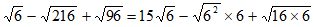[Bring surd in simples form]

=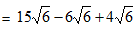= (15 - 6 + 4 )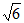=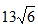Ans.

(ii)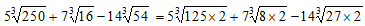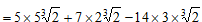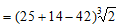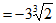Ans.

(iii)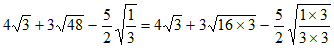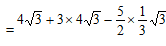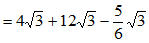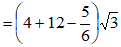=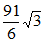Ans.

### MULTIPLICATION AND DIVISION OF SURDS:

e.g. (i)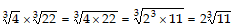(i)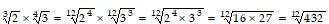### COMPARISON OF SURDS:

It is clear that if x > y > 0 and n > 1 is a positive integer.

e.g. Which is greater is each of the following :

(i)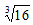and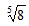(ii)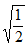and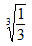L.C.M. of 3 and 5 15. L.C.M. of 2 and 3 is 6.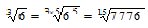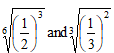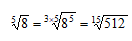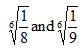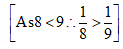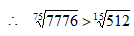so,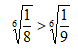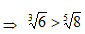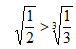### RATIONALIZATION OF SURDS

Rationalizing factor product of two surds is a rational number then each of them is called the rationalizing factor (R.F.) of the other. The process of converting a surd to a rational number by using an appropriate multiplier is known as rationalization.

Some examples:

(i) R.F. of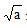is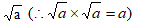(ii) R.F. of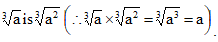.

(iii) R.F. of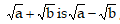& vice versa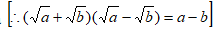.

(iv) R.F. of a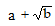is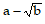& vice versa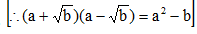(v) R.F. of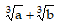is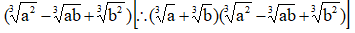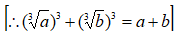which is rational.

(vi) R.F. of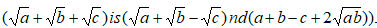question Find the R.G. (rationalizing factor) of the following:

(i)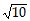(ii)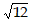(iii)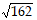(iv)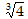(v)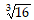(vi)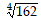(vii)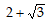(viii)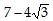(ix)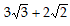(x)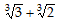(xi)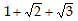Solution: (i).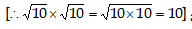as 10 is rational number.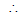R.F. ofisAns.

(ii).First write it’s simplest from i.e.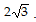.

Now find R.F. (i.e. R.F. of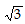is)R.F. of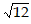isAns.

(iii)Simplest from ofis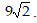.

R.F. of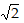is.R.F. ofisAns.